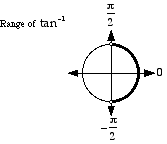index: click on a letter A B C D E F G H I J K L M N O P Q R S T U V W X Y Z A to Z index index: subject areas numbers & symbols sets, logic, proofs geometry algebra trigonometry advanced algebra & pre-calculus calculus advanced topics probability & statistics real world applications multimedia entrieswww.mathwords.com about mathwords website feedback

 Inverse Tangent tan-1 Tan-1 arctan Arctan The inverse function of tangent. Basic idea: To find tan-1 1, we ask "what angle has tangent equal to 1?" The answer is 45°. As a result we say that tan-1 1 = 45°. In radians this is tan-1 1 = π/4. More: There are actually many angles that have tangent equal to 1. We are really asking "what is the simplest, most basic angle that has tangent equal to 1?" As before, the answer is 45°. Thus tan-1 1 = 45° or tan-1 1 = π/4. Details: What is tan-1 (–1)? Do we choose 135°, –45°, 315° , or some other angle? The answer is –45°. With inverse tangent, we select the angle on the right half of the unit circle having measure as close to zero as possible. Thus tan-1 (–1) = –45° or tan-1 (–1) = –π/4. In other words, the range of tan-1 is restricted to (–90°, 90°) or. Note: arctan refers to "arc tangent", or the radian measure of the arc on a circle corresponding to a given value of tangent. Technical note: Since none of the six trig functions sine, cosine, tangent, cosecant, secant, and cotangent are one-to-one, their inverses are not functions. Each trig function can have its domain restricted, however, in order to make its inverse a function. Some mathematicians write these restricted trig functions and their inverses with an initial capital letter (e.g. Tan or Tan-1). However, most mathematicians do not follow this practice. This website does not distinguish between capitalized and uncapitalized trig functions.See also